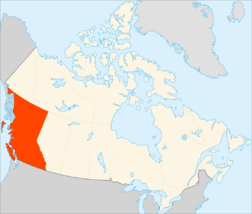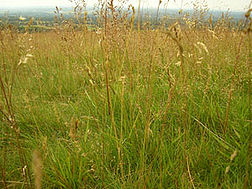# Science:Infinite Series Module/Appendices/The Contrapositive and the Divergence Test/Contrapositive Examples

You are probably familiar with at least a little Canadian geography, and know that British Columbia (BC) is a geographical area within Canada. BC is a province belonging to Canada, as shown in the diagram below.The province of British Columbia (red) is a part of Canada (yellow) (Image courtesy of Wikimedia Commons, public domain).

Consider the statement

If I am standing in BC, then I am standing in Canada.

The contrapositive of this example is

If I am not standing in Canada, then I am not standing in BC.

The contrapositive is certainly true because the entire province of BC is a part of Canada. In fact, the contrapositive is true because the original statement is true: if a part of BC were not in Canada, then both the original statement and the contrapositive would be false.

## Trigonometric Function

Consider the statement

If x is equal to zero, then sin(x) is equal to zero.

This statement is certainly true, and its contrapositive is

If sin(x) is not zero, then x is not zero.

Again, the contrapositive is certainly true. If we take x to be any value so that $\sin(x)$is not zero, then x cannot be zero.

## If the Grass is Not Wet

Suppose we are in an open field of grass.Tall grass in an open field (Image courtesy of Wikimedia Commons, public domain).

Consider the statement

If it is raining, then the grass is wet.

The contrapositive of this example is

If the grass is not wet, then it is not raining.

Sure, the grass could get wet if we were watering the grass. But if the grass is not wet, it can't be raining. Otherwise, the grass would be wet.

## Equivalence

At this point, it may not be clear that in each of the above cases,

• the contrapositive was true because the original statement was true
• the given statement and its contrapositive are equivalent

To get a sense of why this would be so, the next example takes a closer look at the contrapositive.